# Solution in Presence of Resistive Wall

Suppose, now, that the wall at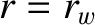possesses non-zero electrical resistivity, but is surrounded by a perfectly conducting wall located at radius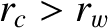. The most general solution to the cylindrical tearing mode equation, (3.60), in the outer region can be written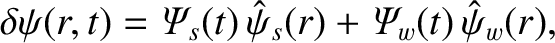(3.76)

where the real function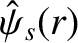is specified in Section 3.8, and the real function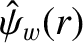is a solution of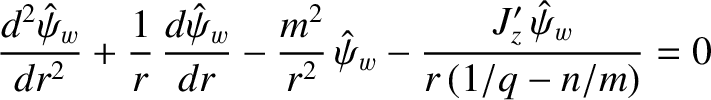(3.77)

that satisfies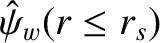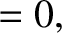(3.78)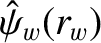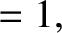(3.79)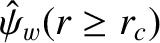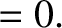(3.80)

Again, Equation (3.80) ensures that the perturbed magnetic field associated with the tearing mode cannot penetrate the perfectly conducting wall. It is easily seen that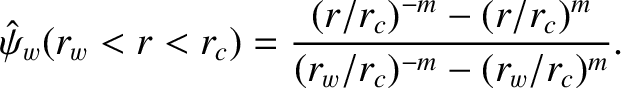(3.81)

In general,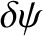is continuous across the resistive wall (in accordance with Maxwell's equations), whereas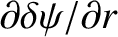is discontinuous. The discontinuity inis caused by a helical current sheet induced in the wall. The complex quantity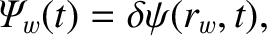(3.82)

determines the amplitude and phase of the perturbed magnetic flux that penetrates the resistive wall. The complex quantity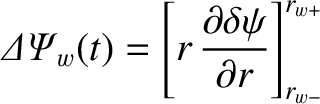(3.83)

parameterizes the amplitude and phase of the helical current sheet flowing in the wall. Simultaneously matching the outer solution (3.76) across the rational surface and the resistive wall yields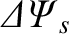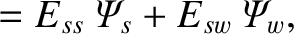(3.84)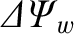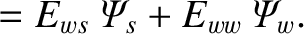(3.85)

Here,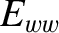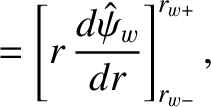(3.86)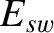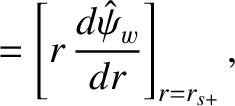(3.87)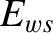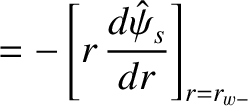(3.88)

are real quantities determined by the solutions of Equations (3.64) and (3.77) in the outer region.

Equations (3.64) and (3.77) can be combined to give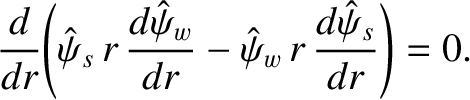(3.89)

If we integrate the previous equation from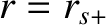to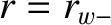, making use of Equations (3.66), (3.67), (3.78), (3.79), (3.87), and (3.88), then we obtain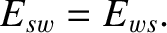(3.90)